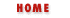### 6.2 Functions as Data Types

The most important features of functions is that they can be defined and invoked, as shown in the previous section. Function definition and invocation are syntactic features of JavaScript, and of most other programming languages. In JavaScript, however, functions are not only syntax, but also data. In some languages, like Java, functions are part of a program, but cannot be manipulated by the program--you cannot, for example, pass one function as an argument to another function in Java. Other languages, like C and C++, are more flexible--while a function defined in C is not actually a data type, "function pointers" can be manipulated by the program, and it is possible to pass these function pointers to other functions and to assign them to variables.

JavaScript goes even further than C. Functions in JavaScript are data, and thus can be treated like any other data value--assigned to variables, stored in the properties of objects or the elements of arrays, passed to functions, and so on. Because JavaScript is an interpreted language, and because it treats functions as a distinct data type, the language (in Navigator 3.0) even allows functions to be defined dynamically, at run-time! We'll see how this is done when we consider the Function object later in this chapter.

We've seen that the function keyword is the syntax used to define a function in a JavaScript program. To understand how functions are JavaScript data as well as JavaScript syntax, we've got to understand what the function keyword really does. function creates a function, as we've seen, but it also defines a variable. In this way, the function keyword is like the var keyword. Consider the following function definition:

```function square(x) { return x*x; }
```
This code does the following:

• Defines a new variable named square.

• Creates a new data value, of type function. This function value expects a single argument named x, and has a body that consists of a single statement: "return x*x;".

• Assigns the newly created function value to the newly defined variable.

When we consider function definition in this light, it becomes clear that the name of a function is really immaterial--it is simply the name of a variable that holds the function. The function can be assigned to another variable, and will still work the same:

```function square(x) { return x*x; }
a = square(4);   // a contains the number 16
b = square;      // now b refers to the same function as square does.
c = b(5);        // c contains the number 25
```
Functions can also be assigned to object properties:

```o = new Object;
o.sq = square;
y = o.sq(16);   // y equals 256
```
Functions don't even require names, as when we assign them to array elements:

```a = new Array(10);
a = square;
a = 20;
a = a(a);  // a contains 400
```
Note that the function invocation syntax in this last example looks strange, but is still a legal use of the JavaScript () operator!

Example 6.2 is a detailed example of the things that can be done when functions are used as data. It demonstrates how functions can be passed as arguments to other functions, and also how they can be stored in associative arrays (which were introduced in Chapter 3, Variables and Data Types, and are explained in detail in Chapter 7, Objects.) This example may be a little tricky, but the comments explain what is going on; it is worth studying carefully.

#### Example 6.2: Using Functions as Data

```// We define some simple functions here
function add(x,y) { return x + y; }
function subtract(x,y) { return x - y; }
function multiply(x,y) { return x * y; }
function divide(x,y) { return x / y; }
// Here's a function that takes one of the above functions
// as an argument and invokes it on two operands
function operate(operator, operand1, operand2)
{
return operator(operand1, operand2);
}
// We could invoke this function like this to compute
// the value (2+3) + (4*5):
// Now we store the functions defined above in an associative array
var operators = new Object();
operators["subtract"] = subtract;
operators["multiply"] = multiply;
operators["divide"] = divide;
operators["pow"] = Math.pow;  // works for predefined functions too.
// This function takes the name of an operator, looks up
// that operator in the array, and then invokes it on the
// supplied operands. Note the syntax used to invoke the
// operator function.
function operate2(op_name, operand1, operand2)
{
if (operators[op_name] == null) return "unknown operator";
else return operators[op_name](operand1, operand2);
}
// We could invoke this function as follows to compute
// the value ("hello" + " " + "world"):Defining and Invoking FunctionsThe Function Object